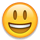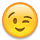# Help needed with customizing Dump

I want to customize Dump for the following class:

```void Main()
{
var list = new List<AggregateRanking> {
new AggregateRanking { A=3, B=0, C=1, D=6},
new AggregateRanking { A=30M, B=0, C=10, D=60, AsPercentage = true },
};

list.Dump(noTotals: true);
}

// You can define other methods, fields, classes and namespaces here
public record AggregateRanking
{
public decimal A { get; set; }
public decimal B { get; set; }
public decimal C { get; set; }
public decimal D { get; set; }

public decimal Total => A + B + C + D;

public bool AsPercentage { get; set; }

object ToDump() => Util.ToExpando(this, exclude: nameof(AsPercentage));
}
```

This currently is dumped as:

AΞΞ BΞΞ CΞΞ DΞΞ TotalΞΞ
3 0 1 6 10
30 0 10 60 100

What I would like to have:

AΞΞ BΞΞ CΞΞ DΞΞ TotalΞΞ
3 0 1 6 10
30 % 0 % 10 % 60 % 100 %

So aligned right and with the percentage sign added if the property `AsPercentage` is true.

How do I do this? Because if I convert the columns to strings, they get aligned to the left.

• For now, you can do the following (although you will lose the auto-graphing option):

```object ToDump()
{
IDictionary<string, object> expando = Util.ToExpando (this, exclude: nameof (AsPercentage));
foreach (var column in expando.ToArray())
if (column.Value is decimal d)
expando [column.Key] = Util.WithStyle (d.ToString() + "%", "float:right");
return expando;
}
```

The next LINQPad beta will allow this (which will preserve the auto-graphing option):

```object ToDump()
{
Util.HtmlHead.AddStyles (".percent::after { content:'%'; }");  // (It's OK to call this multiple times)
IDictionary<string, object> expando = Util.ToExpando (this, exclude: nameof (AsPercentage));
foreach (var column in expando.ToArray())
if (column.Value is decimal d)
expando [column.Key] = Util.WithCssClass (d, "percent");
return expando;
}
```
• Thank you very much!

Changed it a bit:

```    object ToDump()
{
IDictionary<string, object> expando = Util.ToExpando(this, exclude: nameof(AsPercentage));
if (this.AsPercentage)
{
foreach (var column in expando.ToArray())
{
if (column.Value is decimal d)
{
expando[column.Key] = Util.WithStyle(d.ToString() + " %", "float:right");
}
}
}
return expando;
}
```

It now does exactly what I wanted.Only the line

```IDictionary<string, object> expando = Util.ToExpando(this, exclude: nameof(AsPercentage));
```

gives a warning:
Warning: CS8619 Nullability of reference types in value of type 'ExpandoObject' doesn't match target type 'IDictionary<string, object>'.

For now I ignore it...• If you have nullable reference types enabled, change `IDictionary<string,object>` to `IDictionary<string,object?>` to avoid the warning.